# 高品质后处理：十种图像模糊算法的总结与实现

04/07 13:15

本文由@浅墨_毛星云 出品，转载请注明出处

CSDN版文章链接： https://qianmo.blog.csdn.net/article/details/105350519

知乎专栏版文章链接：https://zhuanlan.zhihu.com/p/125744132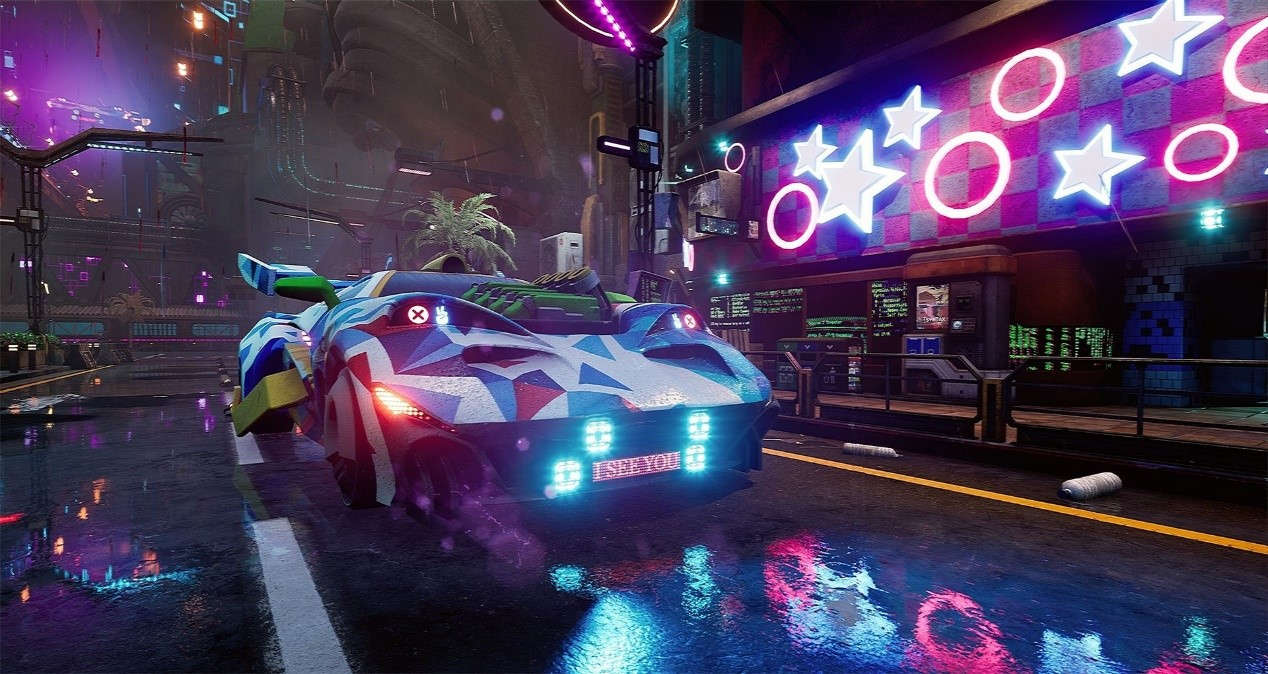• 高斯模糊（Gaussian Blur）

• 方框模糊（Box Blur）

• Kawase模糊（Kawase Blur）

• 双重模糊（Dual Blur）

• 散景模糊（Bokeh Blur）

• 移轴模糊（Tilt Shift Blur）

• 光圈模糊（Iris Blur）

• 粒状模糊（Grainy Blur）

• 方向模糊（Directional Blur）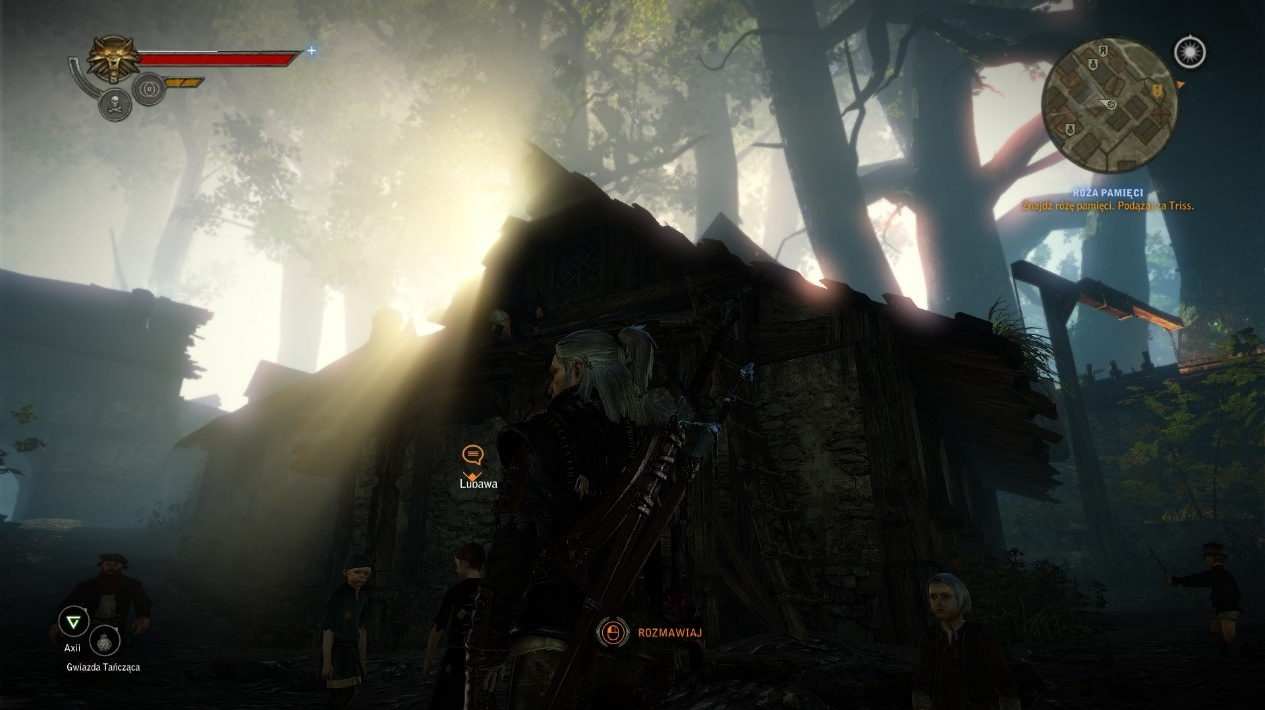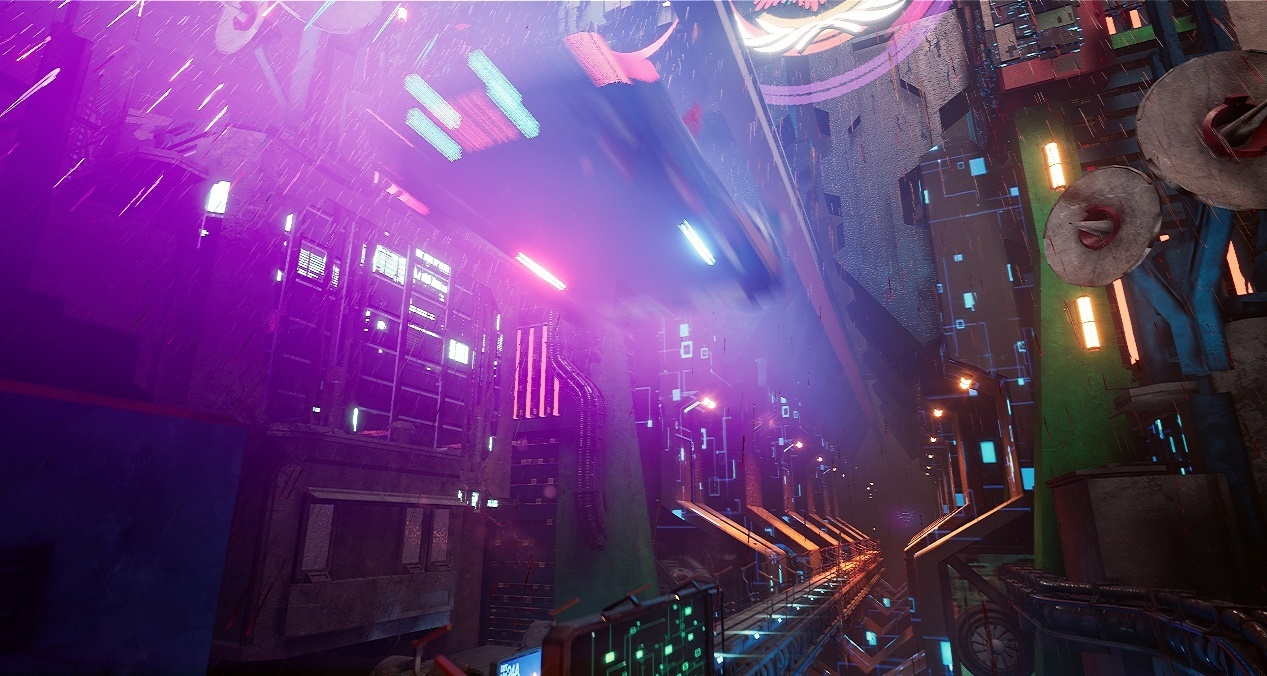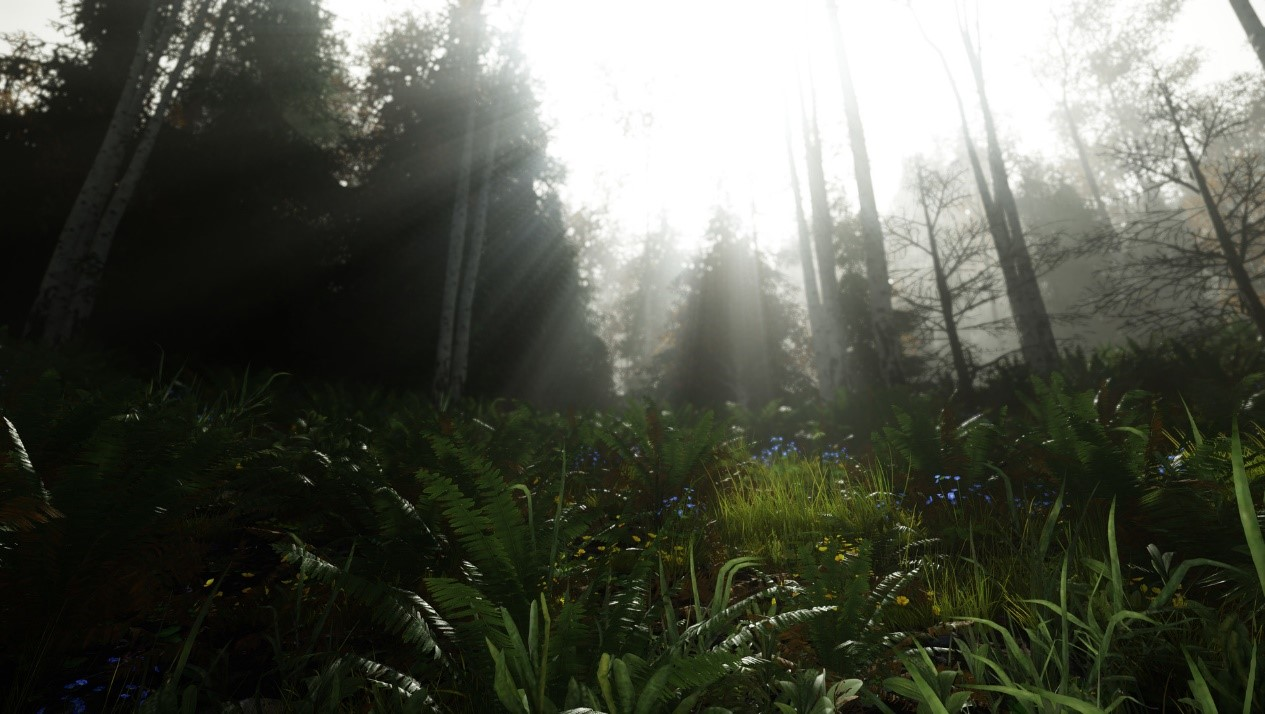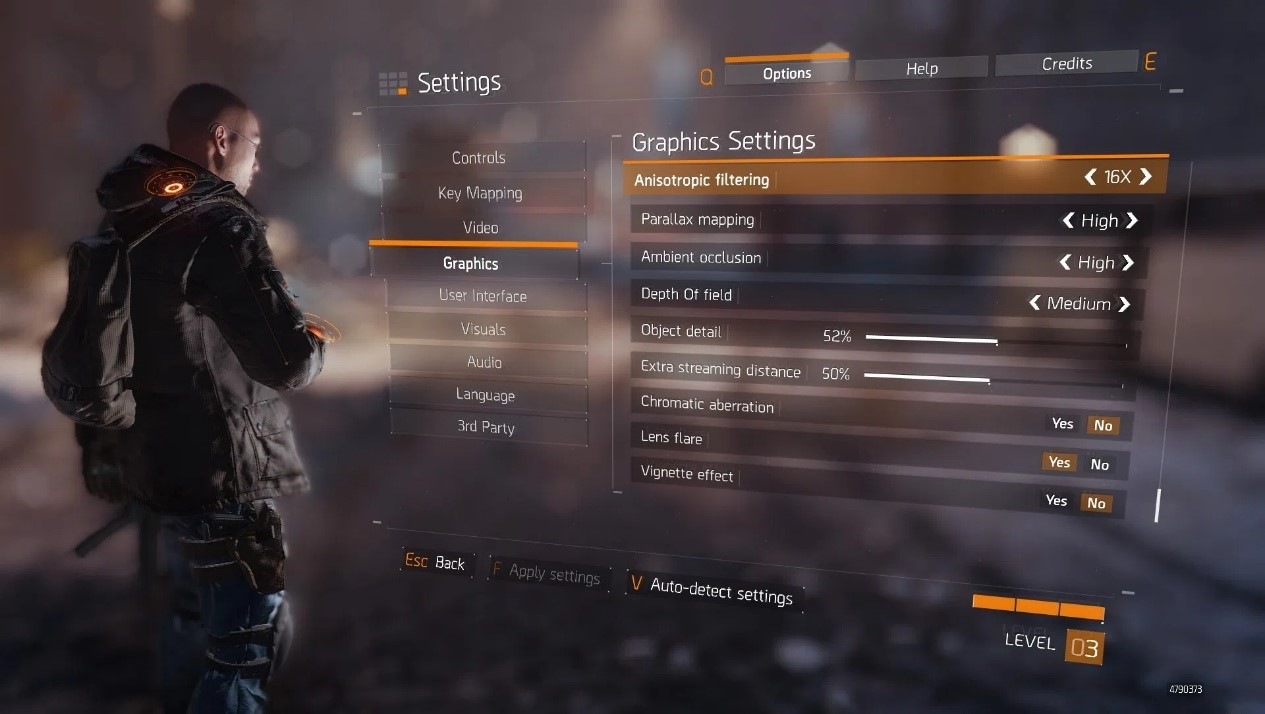其中对于赛博朋克风夜中国风城市的展现，如果缺少了Bloom和Glare Lens Flare等依赖于本文讲到的模糊算法的后处理特效，展现出来的品质将少了很多韵味：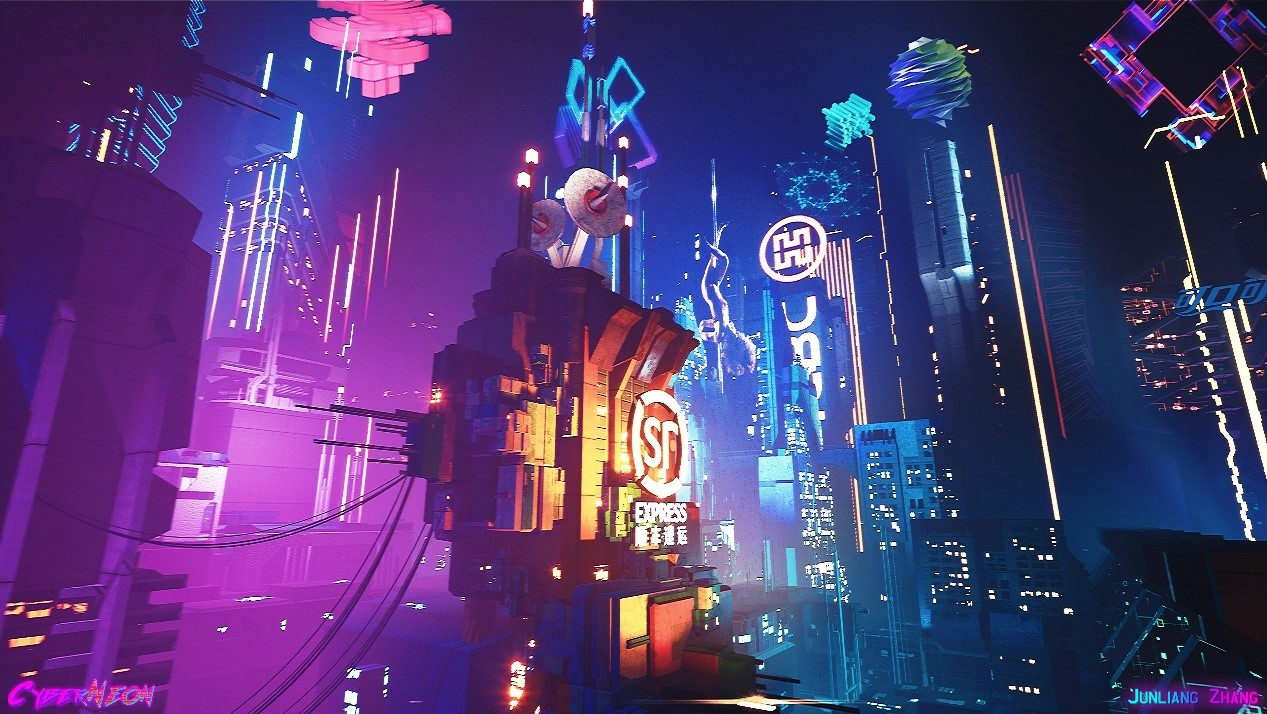# 十种图像模糊算法横向对比

• 模糊品质（Quality） 。模糊品质的好坏是模糊算法是否优秀的主要指标。

• 模糊稳定性（Stability） 。模糊的稳定性决定了在画面变化过程中，模糊是否稳定，不会出现跳变或者闪烁。

• 性能（Performance） 。性能的好坏是模糊算法是否能被广泛使用的关键所在。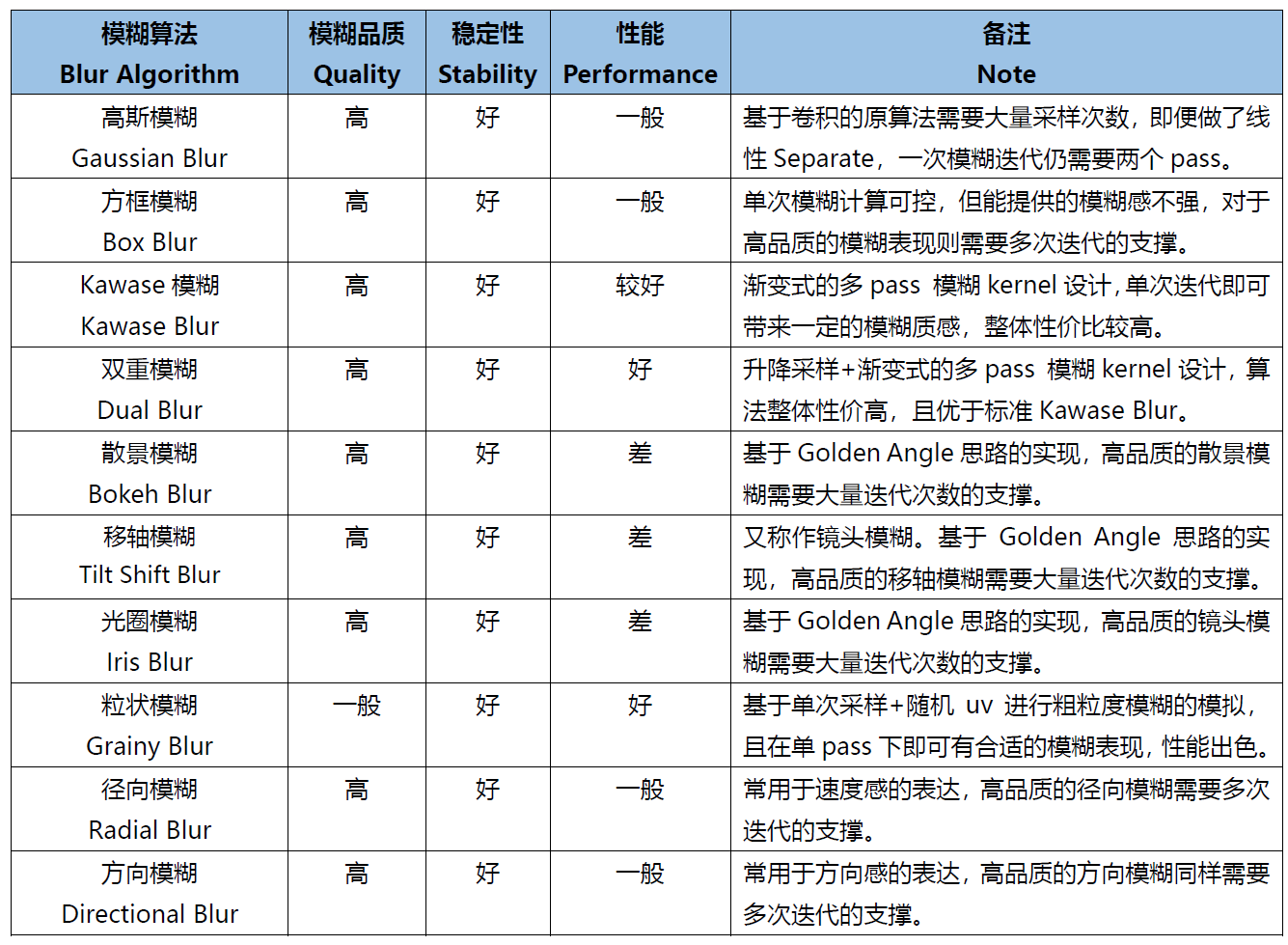# 关于X-PostProcessing Libray

X-PostProcessing Libray，简称XPL，是本人开发的Unity引擎下的高品质开源后处理算法库，旨在提供业界主流的高品质后处理特效的完整解决方案，目前已完美支持Unity Post-processing Stack v2。后续也将提供对Unity引擎URP/LWRP/HDRP的兼容支持。OK，下面我们开始正文。先从最热门，最为大众所熟知的高斯模糊开始。

# 一、高斯模糊（Gaussian Blur）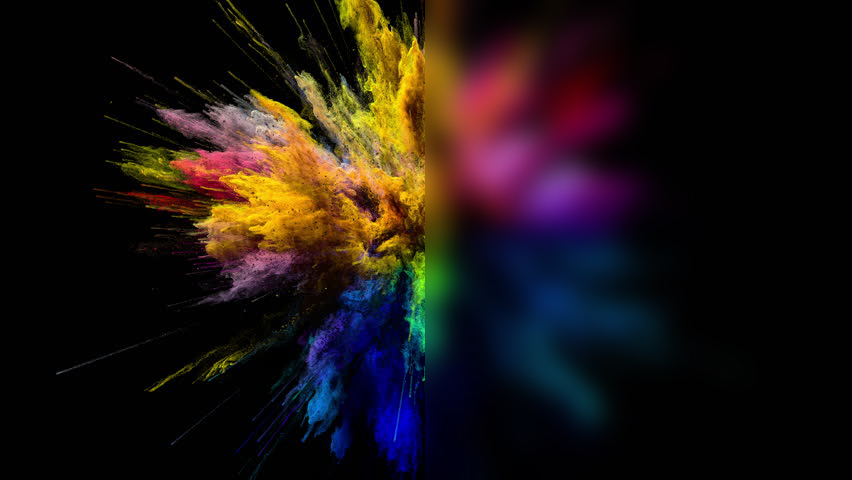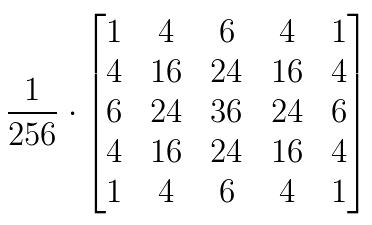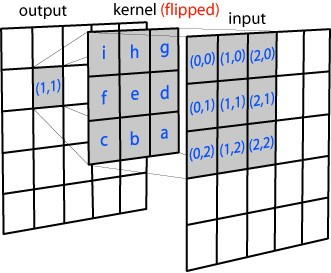N维空间高斯模糊方程可以表示为：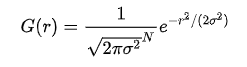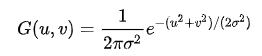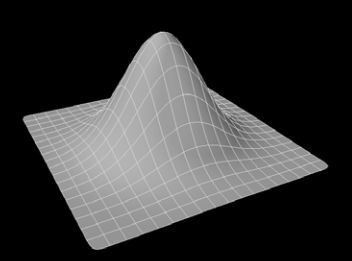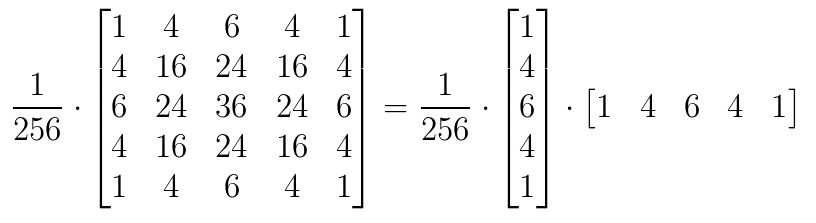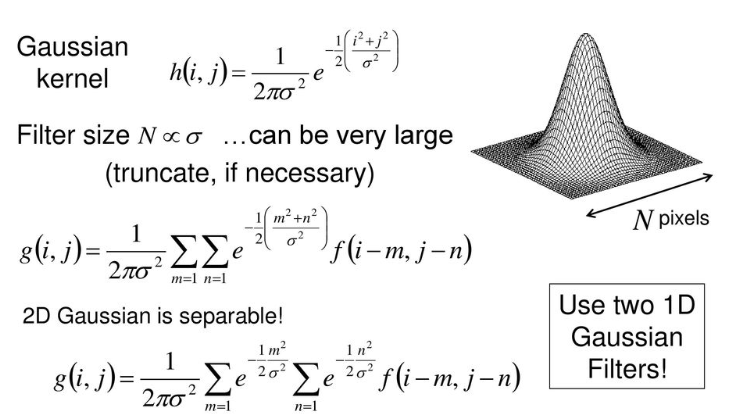``````float4 FragGaussianBlur(v2f i): SV_Target
{
half4 color = float4(0, 0, 0, 0);

color += 0.40 * SAMPLE_TEXTURE2D(_MainTex, sampler_MainTex, i.uv);
color += 0.15 * SAMPLE_TEXTURE2D(_MainTex, sampler_MainTex, i.uv01.xy);
color += 0.15 * SAMPLE_TEXTURE2D(_MainTex, sampler_MainTex, i.uv01.zw);
color += 0.10 * SAMPLE_TEXTURE2D(_MainTex, sampler_MainTex, i.uv23.xy);
color += 0.10 * SAMPLE_TEXTURE2D(_MainTex, sampler_MainTex, i.uv23.zw);
color += 0.05 * SAMPLE_TEXTURE2D(_MainTex, sampler_MainTex, i.uv45.xy);
color += 0.05 * SAMPLE_TEXTURE2D(_MainTex, sampler_MainTex, i.uv45.zw);

return color;
}
``````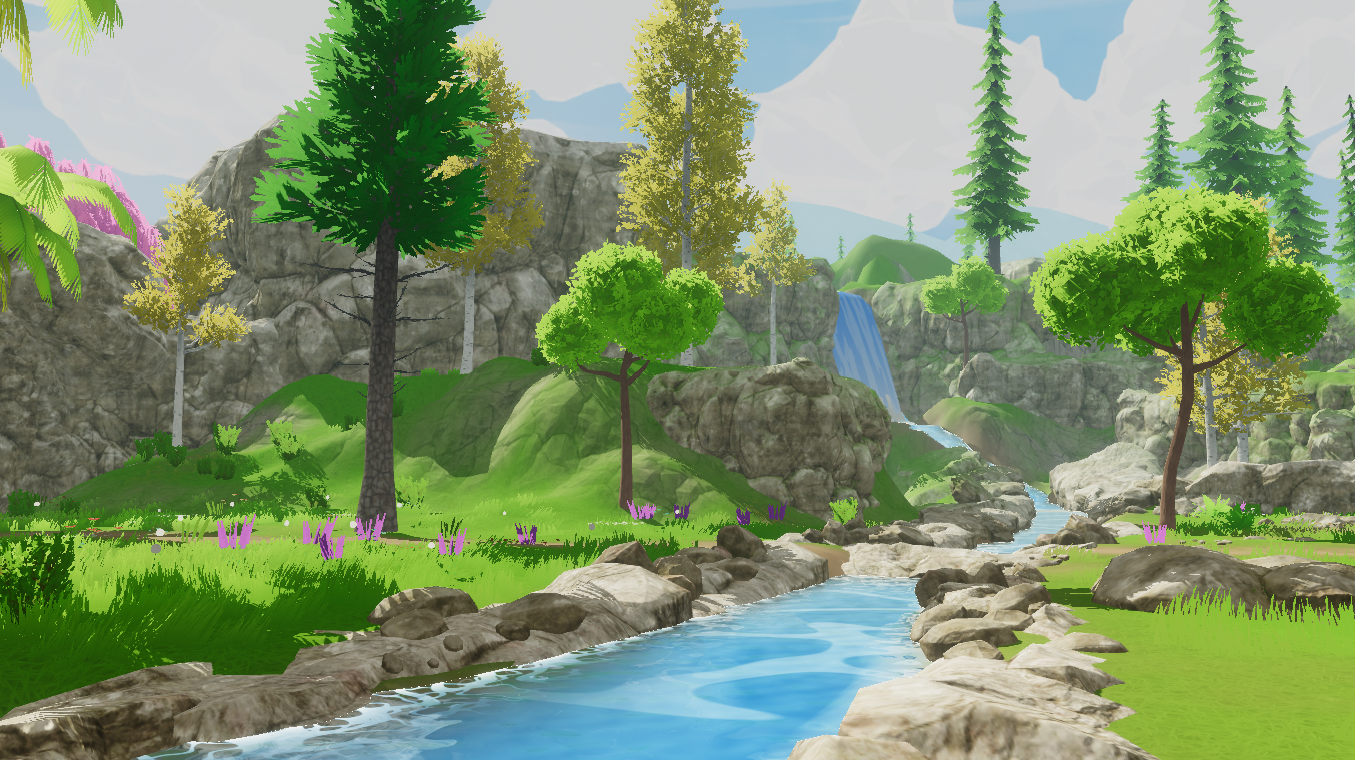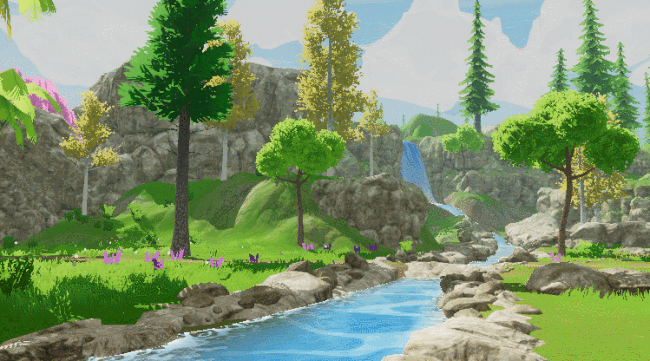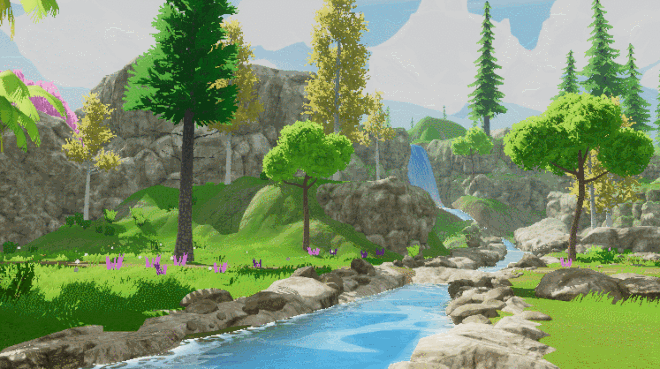# 二、方框模糊（Box Blur）

Box Blur和高斯模糊的性质对比可见下图：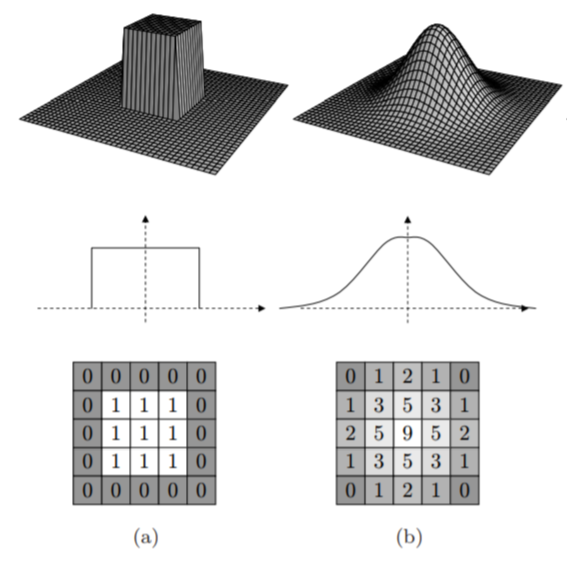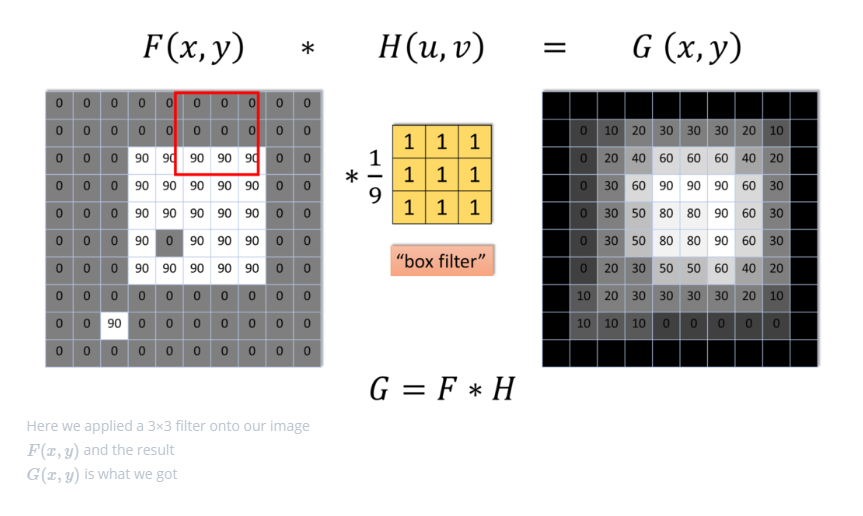Box Blur也是线性可分的，如有需要，也可以借助其此性质，如下所示：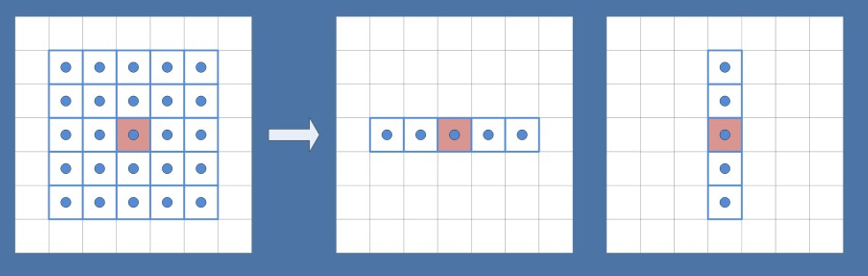``````half4 BoxFilter_4Tap(TEXTURE2D_ARGS(tex, samplerTex), float2 uv, float2 texelSize)
{
float4 d = texelSize.xyxy * float4(-1.0, -1.0, 1.0, 1.0);

half4 s = 0;
s = SAMPLE_TEXTURE2D(tex, samplerTex, uv + d.xy) * 0.25h;  // 1 MUL
s += SAMPLE_TEXTURE2D(tex, samplerTex, uv + d.zy) * 0.25h; // 1 MAD
s += SAMPLE_TEXTURE2D(tex, samplerTex, uv + d.xw) * 0.25h; // 1 MAD
s += SAMPLE_TEXTURE2D(tex, samplerTex, uv + d.zw) * 0.25h; // 1 MAD

return s;
}
``````

Box Blur的渲染效果接近高斯模糊，但性价比并不高，需要较多的迭代次数才能达到高品质的模糊效果：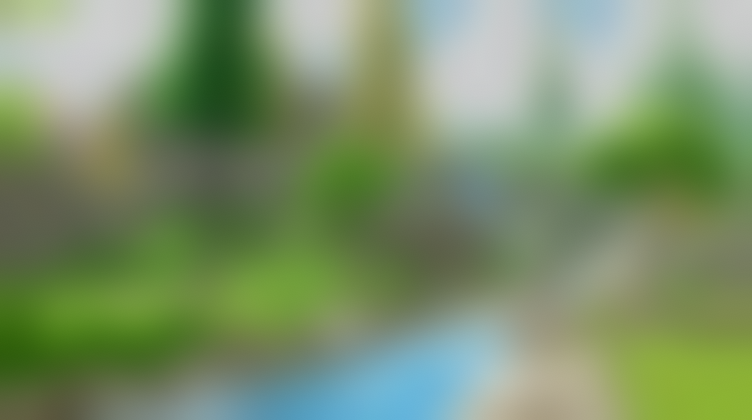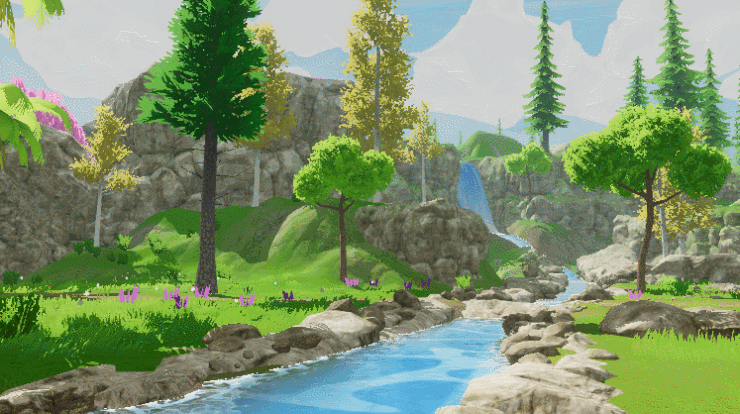# 三、Kawase模糊（Kawase Blur）

Kawase Blur于Masaki Kawase 在GDC2003的分享《Frame Buffer Postprocessing Effects in DOUBLE-S.T.E.A.L (Wreckless)》中提出。Kawase Blur最初用于Bloom后处理特效，但其可以推广作为专门的模糊算法使用，且在模糊外观表现上与高斯模糊非常接近。 Kawase Blur的思路是对距离当前像素越来越远的地方对四个角进行采样，且在两个大小相等的纹理之间进行乒乓式的blit。创新点在于，采用了随迭代次数移动的blur kernel，而不是类似高斯模糊，或box blur一样从头到尾固定的blur kernel。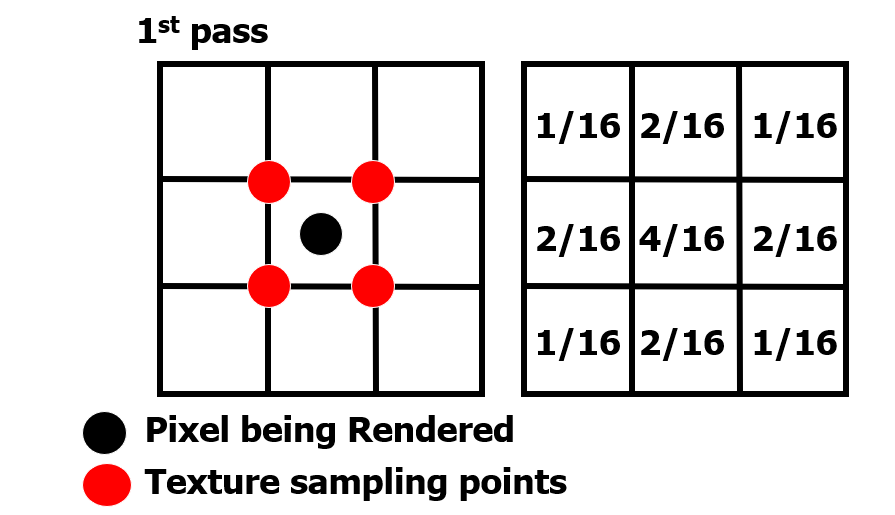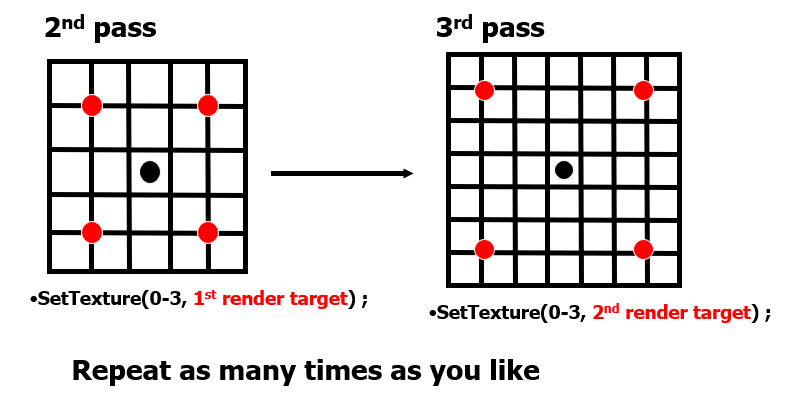``````half4 KawaseBlur(TEXTURE2D_ARGS(tex, samplerTex), float2 uv, float2 texelSize, half pixelOffset)
{
half4 o = 0;
o += SAMPLE_TEXTURE2D(tex, samplerTex, uv + float2(pixelOffset +0.5, pixelOffset +0.5) * texelSize);
o += SAMPLE_TEXTURE2D(tex, samplerTex, uv + float2(-pixelOffset -0.5, pixelOffset +0.5) * texelSize);
o += SAMPLE_TEXTURE2D(tex, samplerTex, uv + float2(-pixelOffset -0.5, -pixelOffset -0.5) * texelSize);
o += SAMPLE_TEXTURE2D(tex, samplerTex, uv + float2(pixelOffset +0.5, -pixelOffset -0.5) * texelSize);
return o * 0.25;
}
``````

Kawase Blur渲染效果接近高斯模糊，但具有更好的性能：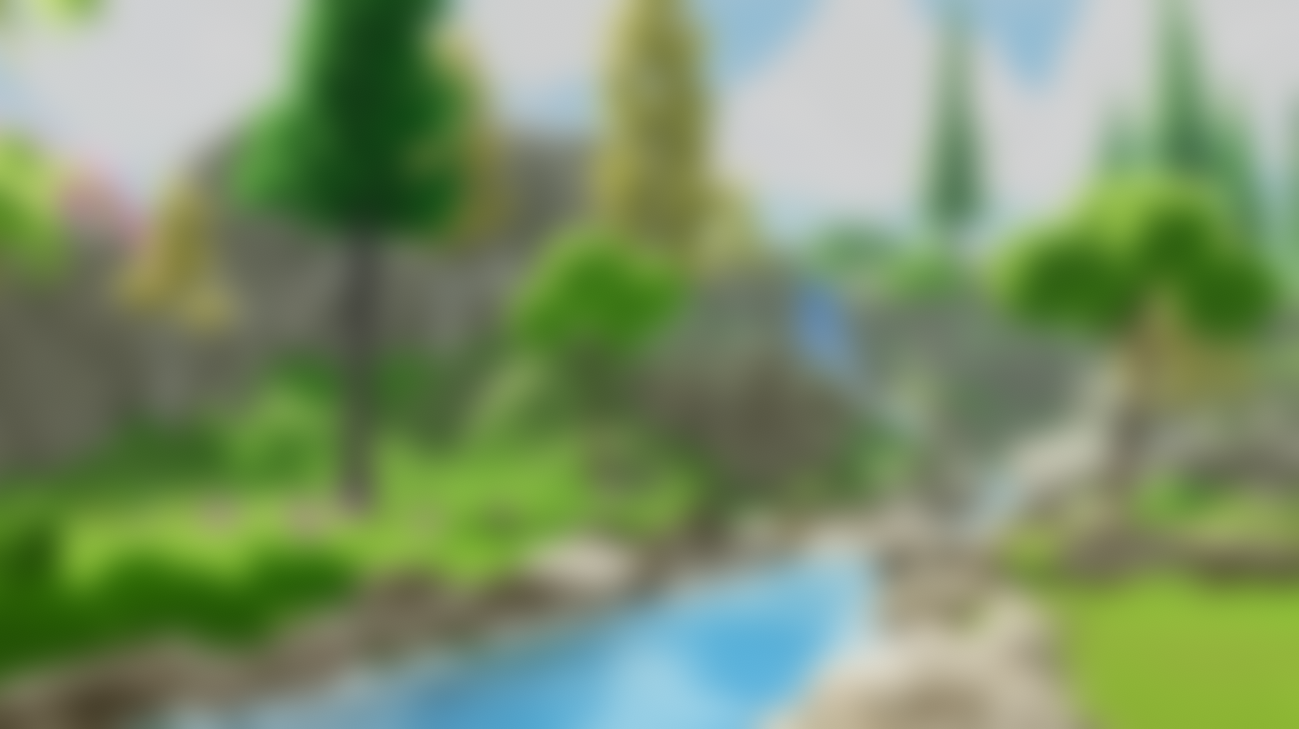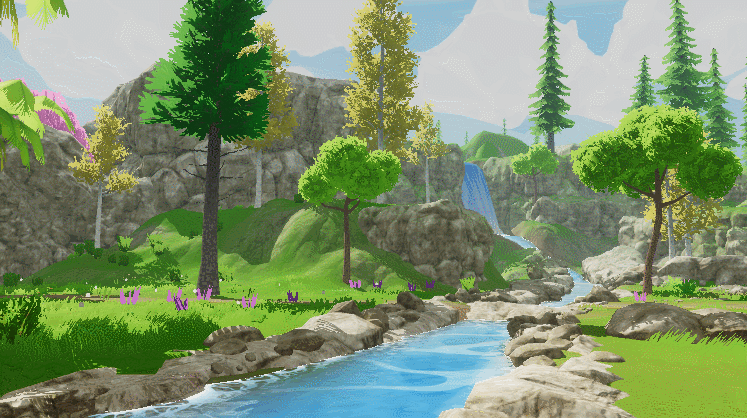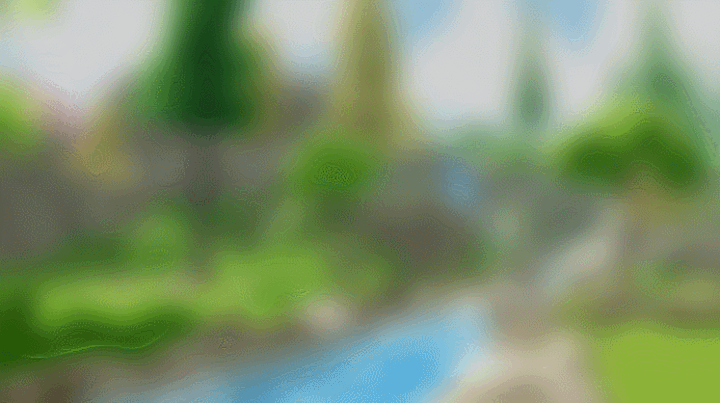# 四、双重模糊（Dual Blur）

Dual Kawase Blur，简称Dual Blur，是SIGGRAPH 2015上ARM团队提出的一种衍生自Kawase Blur的模糊算法。其由两种不同的Blur Kernel构成，如下图所示。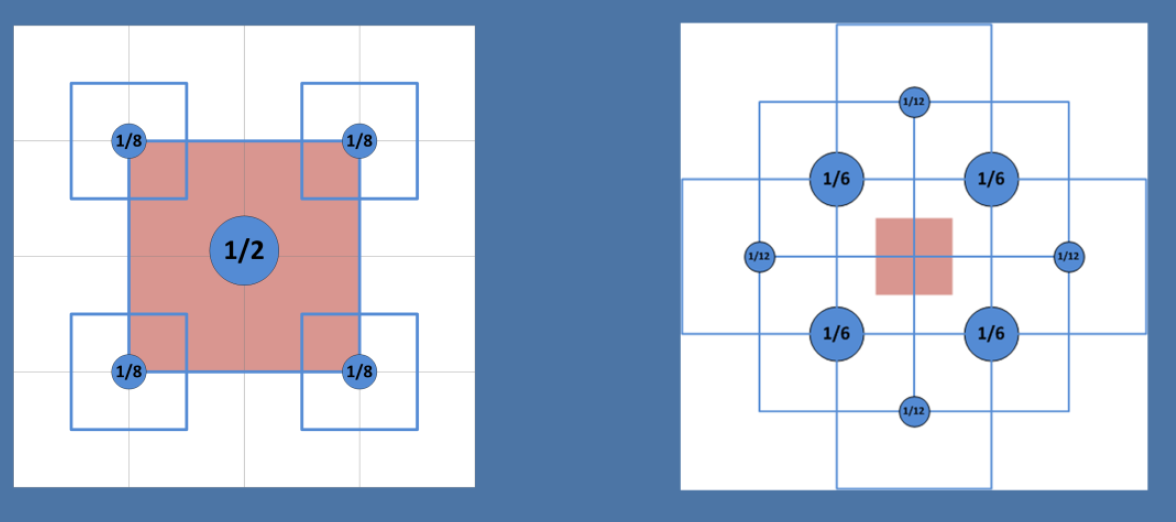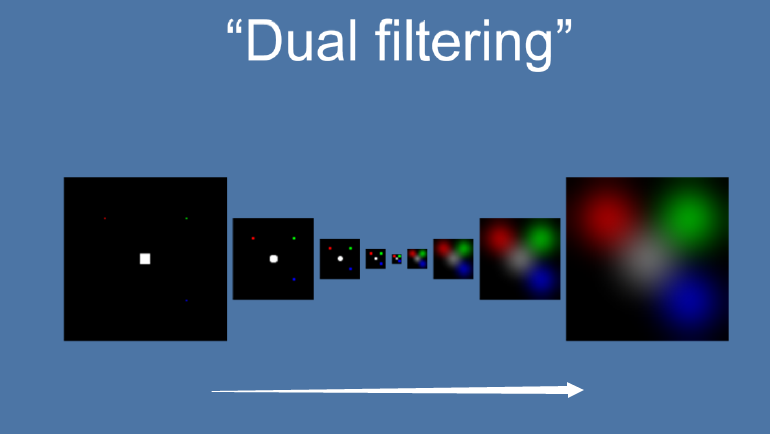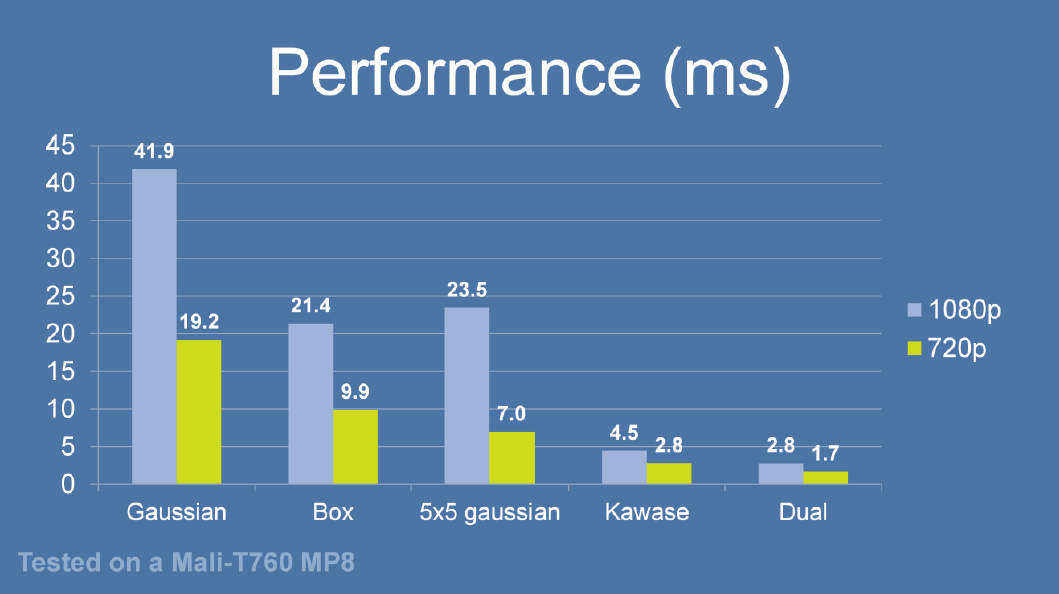``````half4 Frag_DownSample(v2f_DownSample i): SV_Target
{
half4 sum = SAMPLE_TEXTURE2D(_MainTex, sampler_MainTex, i.uv) * 4;
sum += SAMPLE_TEXTURE2D(_MainTex, sampler_MainTex, i.uv01.xy);
sum += SAMPLE_TEXTURE2D(_MainTex, sampler_MainTex, i.uv01.zw);
sum += SAMPLE_TEXTURE2D(_MainTex, sampler_MainTex, i.uv23.xy);
sum += SAMPLE_TEXTURE2D(_MainTex, sampler_MainTex, i.uv23.zw);

return sum * 0.125;
}

half4 Frag_UpSample(v2f_UpSample i): SV_Target
{
half4 sum = 0;
sum += SAMPLE_TEXTURE2D(_MainTex, sampler_MainTex, i.uv01.xy);
sum += SAMPLE_TEXTURE2D(_MainTex, sampler_MainTex, i.uv01.zw) * 2;
sum += SAMPLE_TEXTURE2D(_MainTex, sampler_MainTex, i.uv23.xy);
sum += SAMPLE_TEXTURE2D(_MainTex, sampler_MainTex, i.uv23.zw) * 2;
sum += SAMPLE_TEXTURE2D(_MainTex, sampler_MainTex, i.uv45.xy);
sum += SAMPLE_TEXTURE2D(_MainTex, sampler_MainTex, i.uv45.zw) * 2;
sum += SAMPLE_TEXTURE2D(_MainTex, sampler_MainTex, i.uv67.xy);
sum += SAMPLE_TEXTURE2D(_MainTex, sampler_MainTex, i.uv67.zw) * 2;

return sum * 0.0833;
}
``````

XPL中也提供了启发自Dual Kawase Blur的Dual Gaussian Blur、Dual Box Blur、Dual Tent Blur的实现。

Dual Kawase Blur最终的模糊效果截图如下，可以看到其与高斯模糊的模糊表现也非常接近：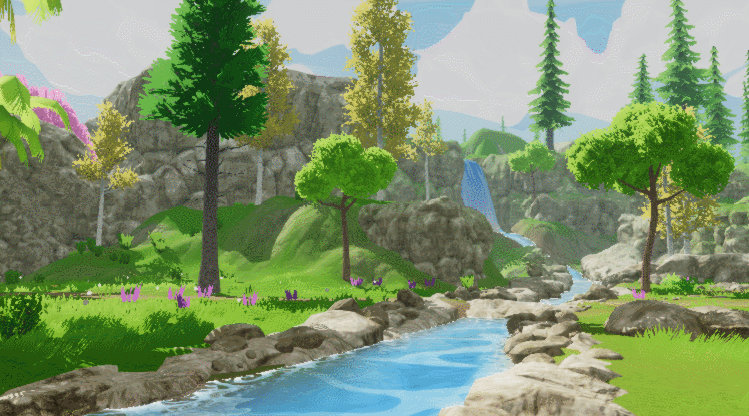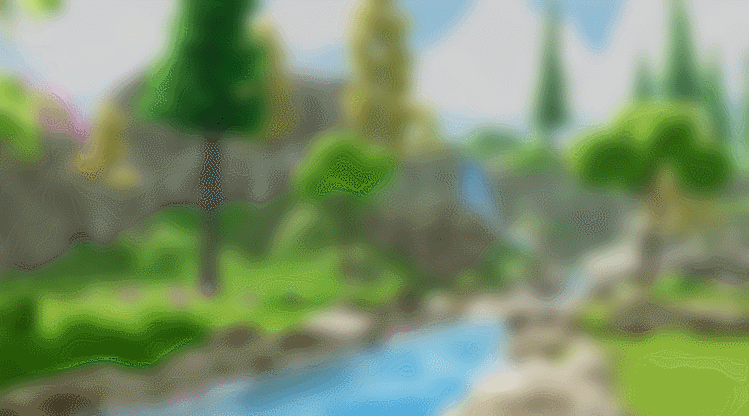# 五、散景模糊（Bokeh Blur）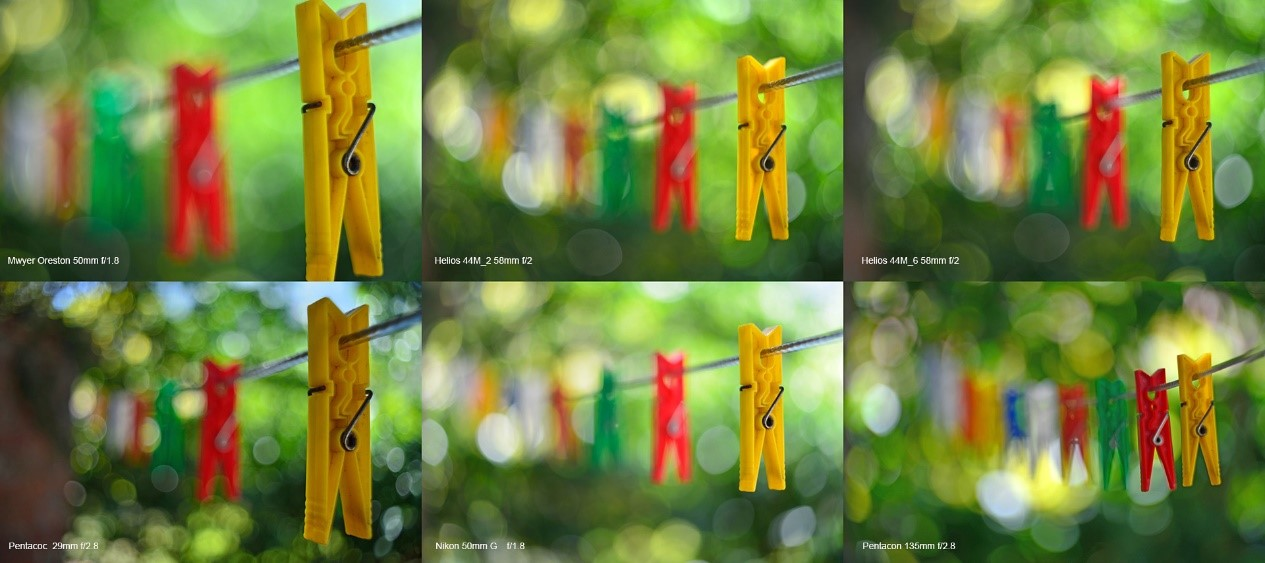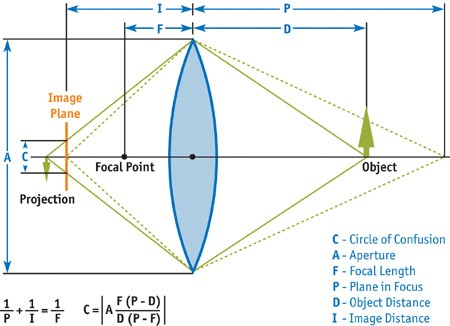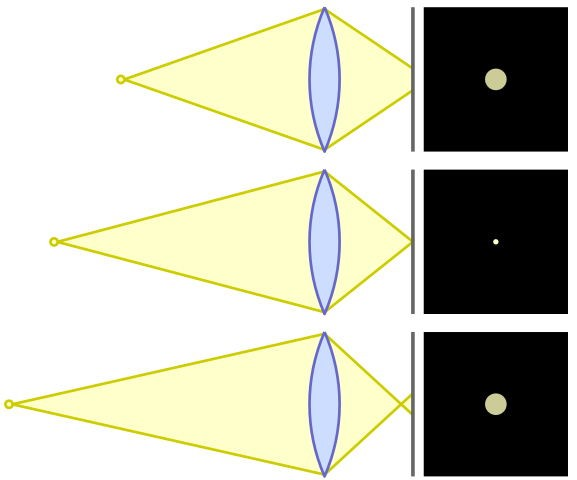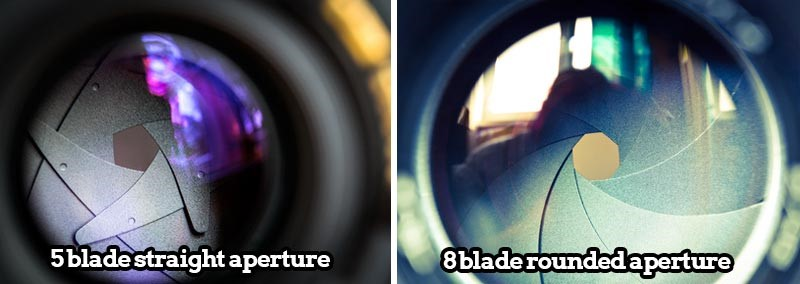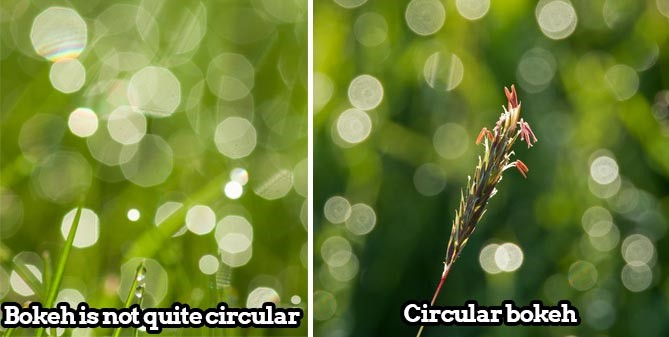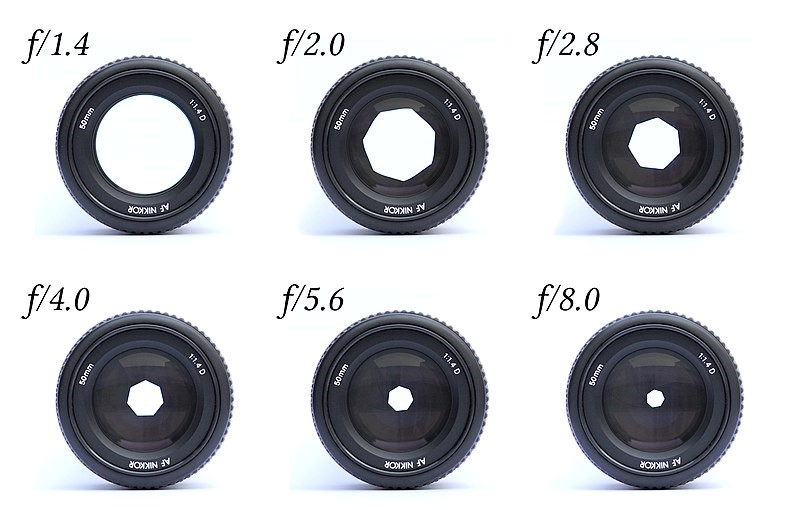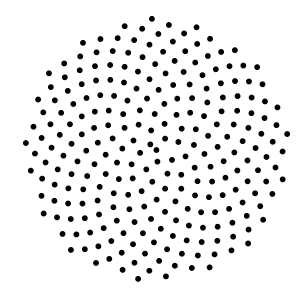``````half4 BokehBlur(VaryingsDefault i)
{
half2x2 rot = half2x2(_GoldenRot);
half4 accumulator = 0.0;
half4 divisor = 0.0;

half r = 1.0;

for (int j = 0; j < _Iteration; j++)
{
r += 1.0 / r;
angle = mul(rot, angle);
half4 bokeh = SAMPLE_TEXTURE2D(_MainTex, sampler_MainTex, float2(i.texcoord + _PixelSize * (r - 1.0) * angle));
accumulator += bokeh * bokeh;
divisor += bokeh;
}
return accumulator / divisor;
}
``````

https://github.com/QianMo/X-PostProcessing-Library/tree/master/Assets/X-PostProcessing/Effects/BokehBlur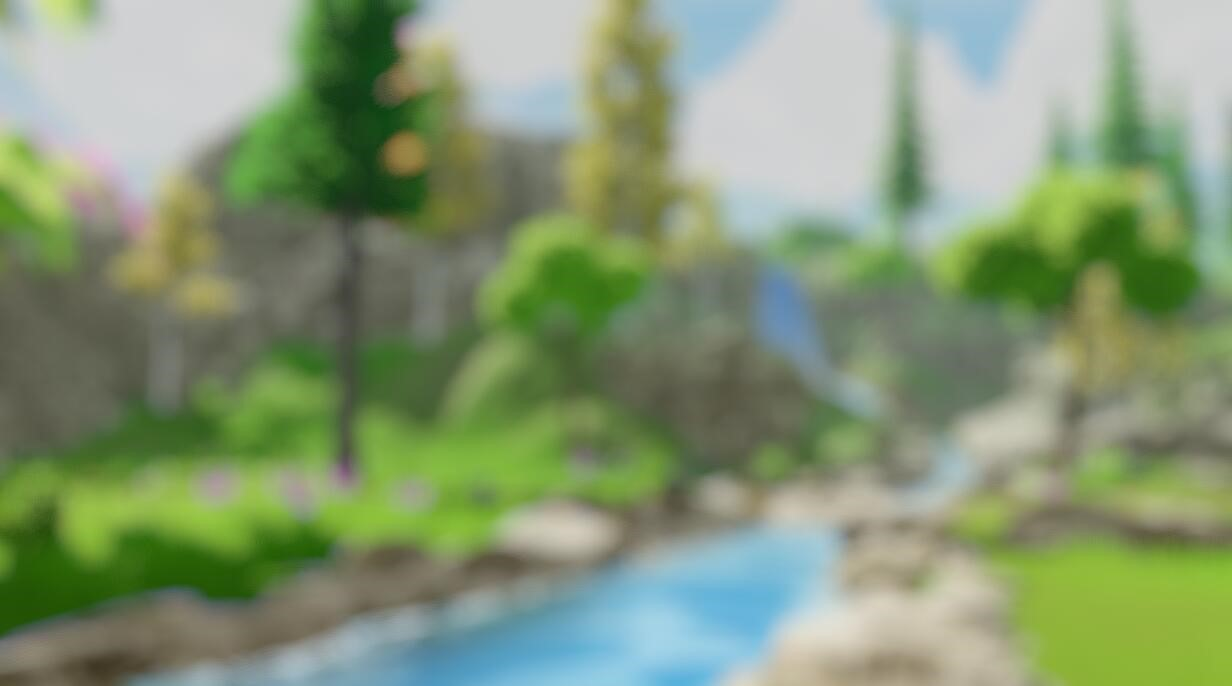# 六、移轴模糊 （Tilt Shift Blur）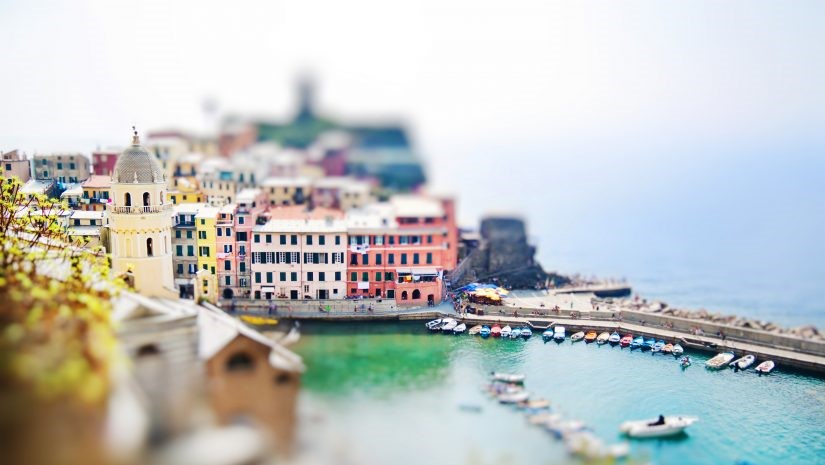``````float TiltShiftMask(float2 uv)
{
float centerY = uv.y * 2.0 - 1.0 + _Offset; // [0,1] -> [-1,1]
}
``````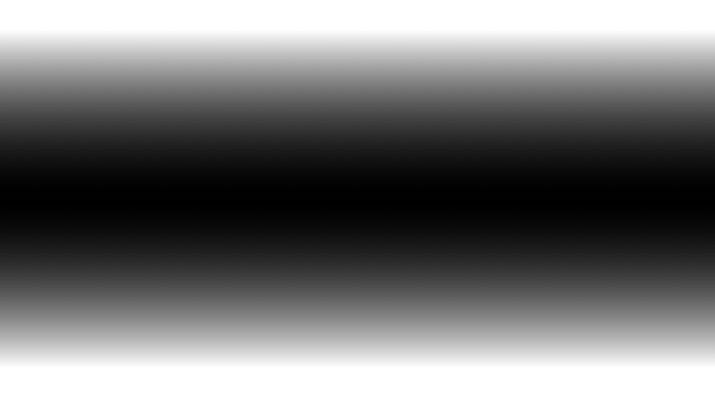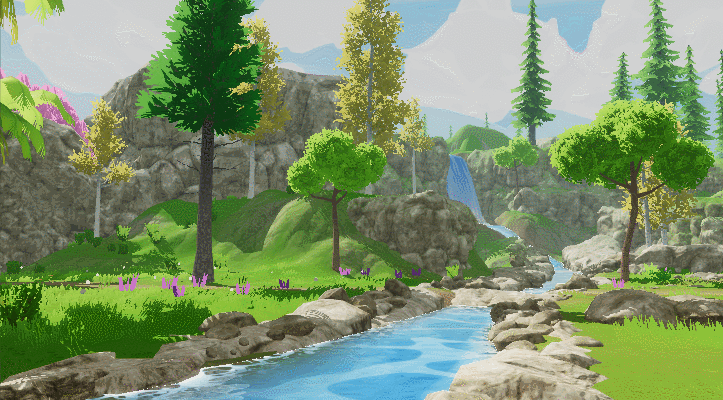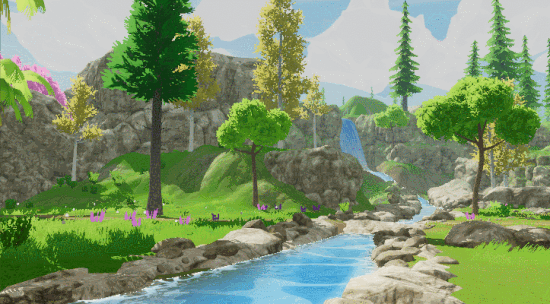# 七、光圈模糊（Iris Blur）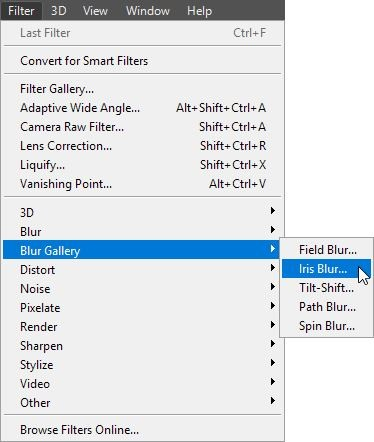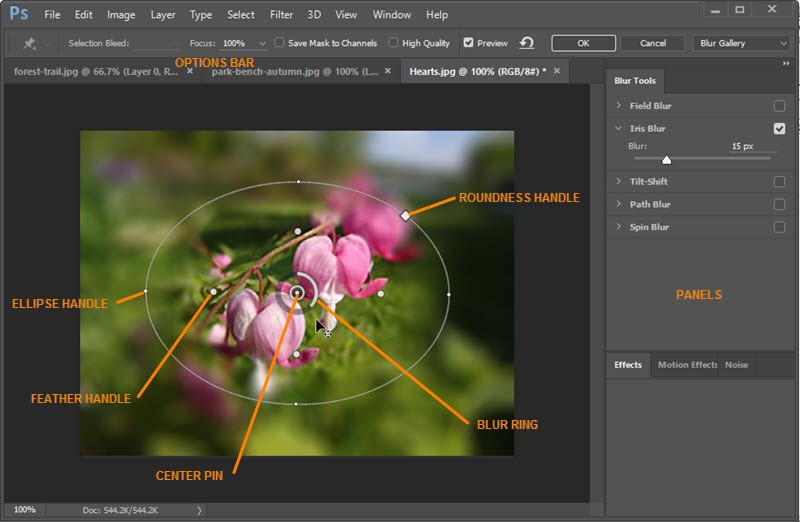``````float IrisMask(float2 uv)
{
float2 center = uv * 2.0 - 1.0 + _Offset; // [0,1] -> [-1,1]
return dot(center, center) * _AreaSize;
}
``````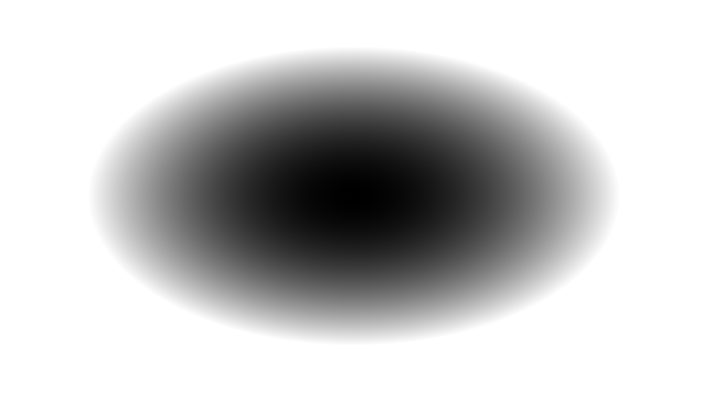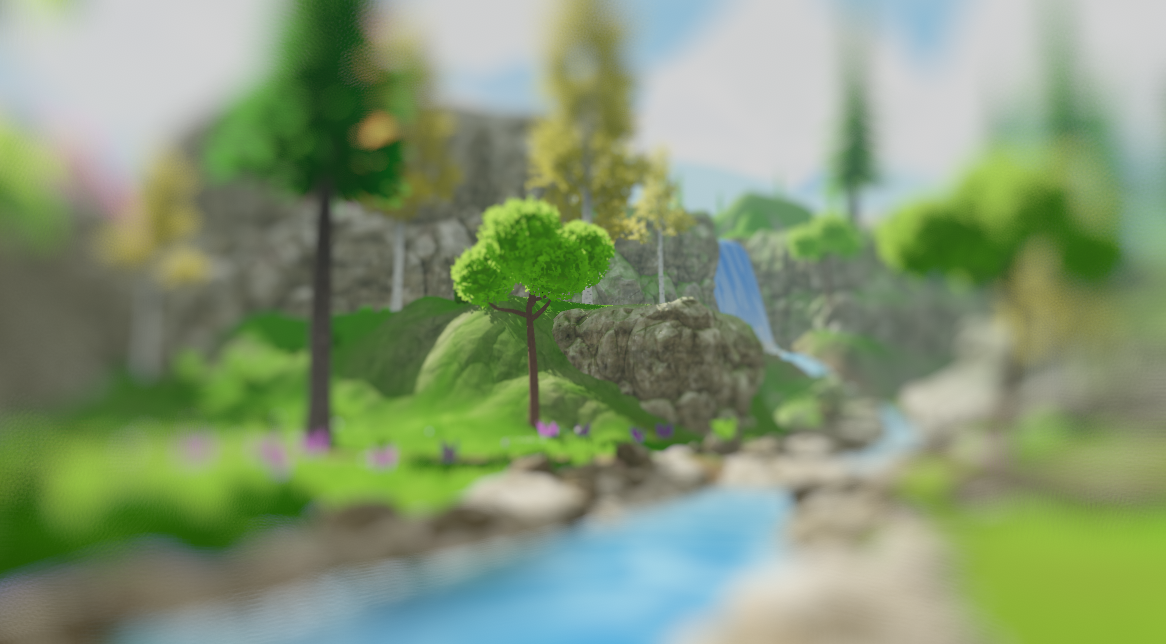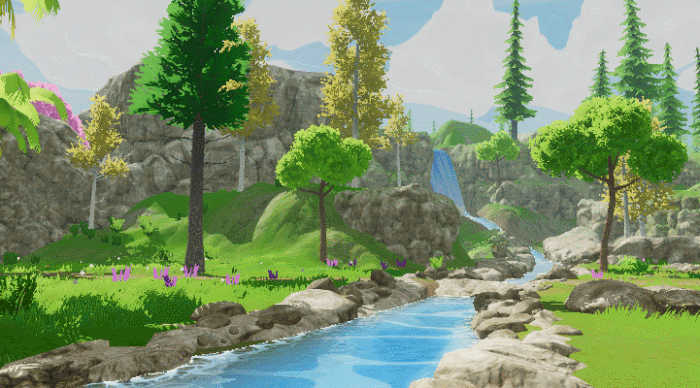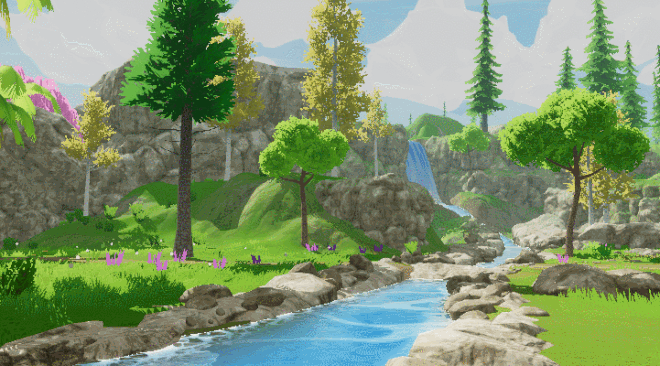# 八、粒状模糊（Grainy Blur）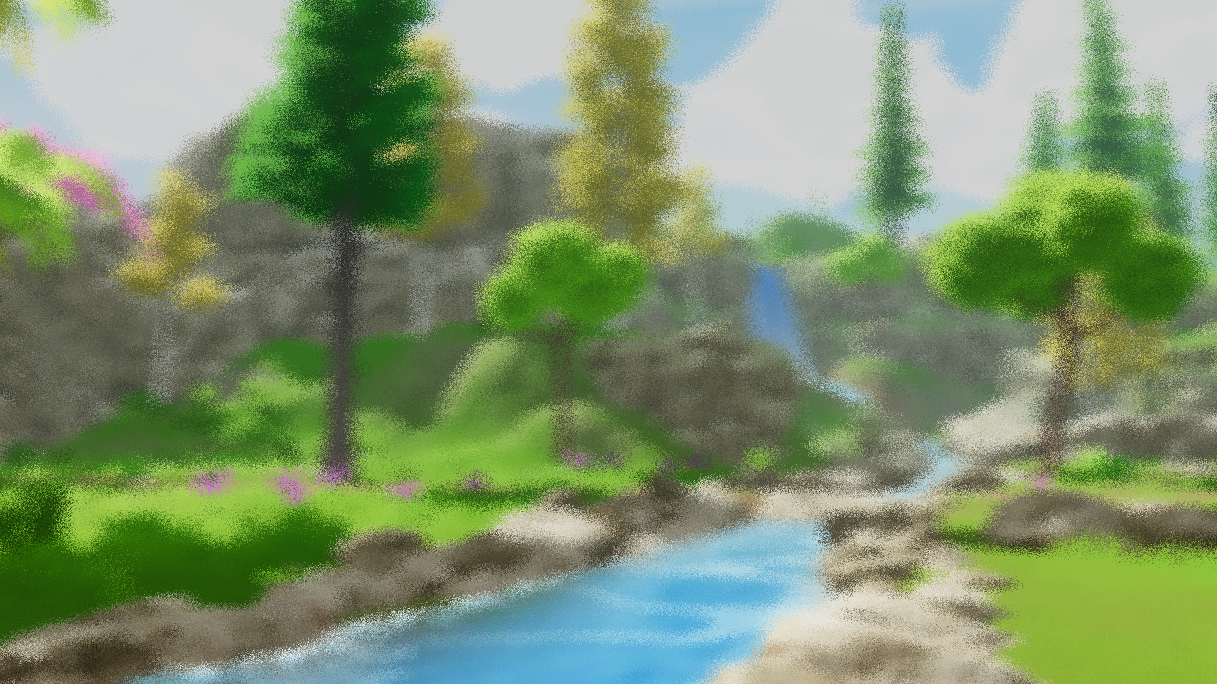``````float Rand(float2 n)
{
return sin(dot(n, half2(1233.224, 1743.335)));
}

half4 GrainyBlur(VaryingsDefault i)
{
half2 randomOffset = float2(0.0, 0.0);
half4 finalColor = half4(0.0, 0.0, 0.0, 0.0);
float random = Rand(i.texcoord);

for (int k = 0; k < int(_Iteration); k ++)
{
random = frac(43758.5453 * random + 0.61432);;
randomOffset.x = (random - 0.5) * 2.0;
random = frac(43758.5453 * random + 0.61432);
randomOffset.y = (random - 0.5) * 2.0;

finalColor += SAMPLE_TEXTURE2D(_MainTex, sampler_MainTex, half2(i.texcoord + randomOffset * _BlurRadius));
}
return finalColor / _Iteration;
}
``````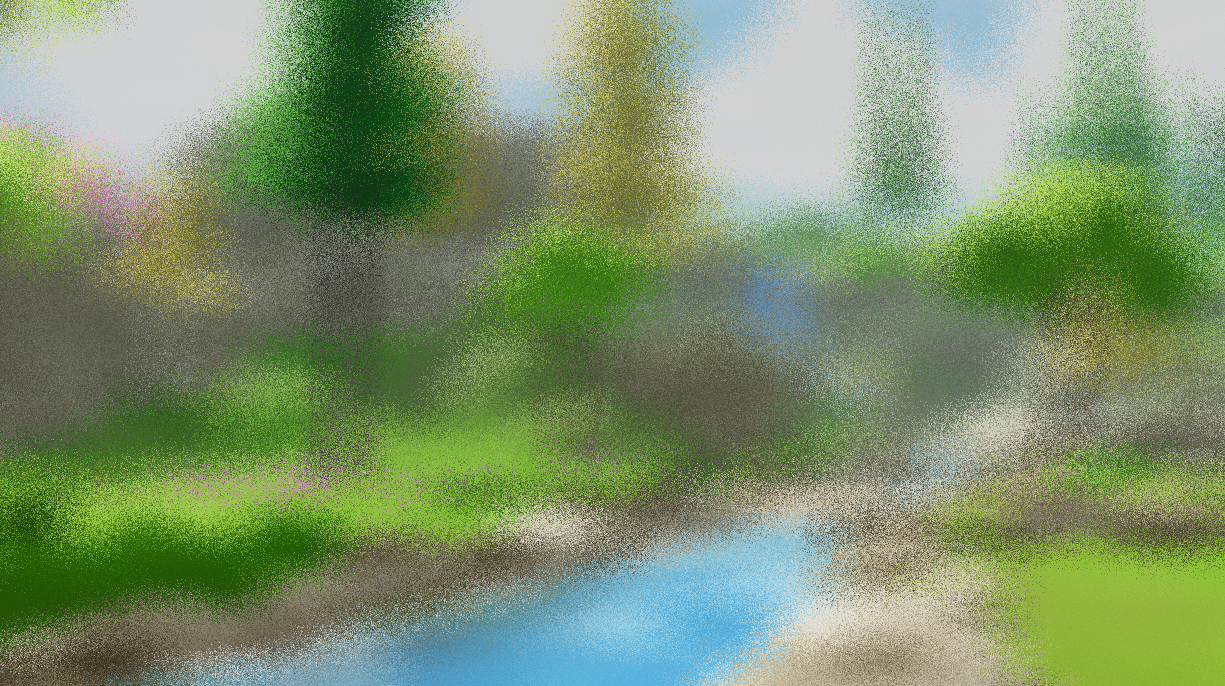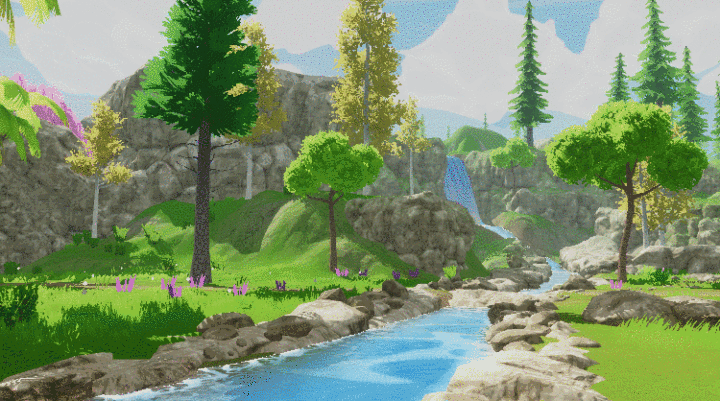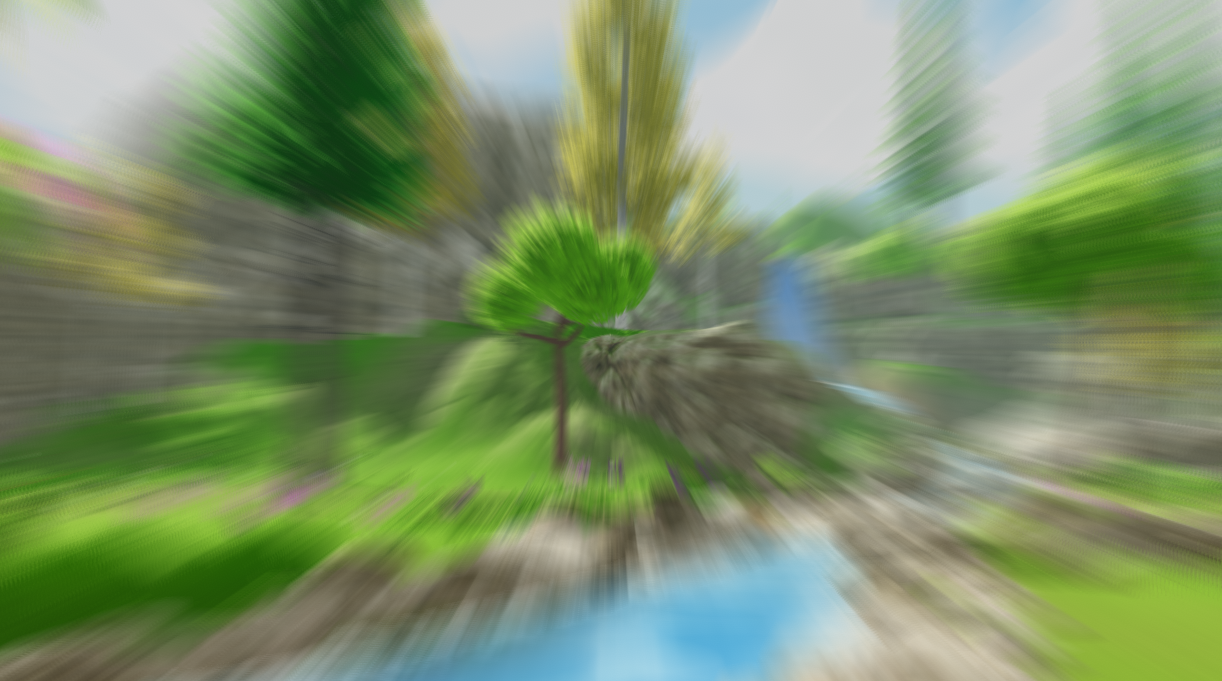``````half4 RadialBlur(VaryingsDefault i)
{

half4 acumulateColor = half4(0, 0, 0, 0);

[unroll(30)]
for (int j = 0; j < _Iteration; j ++)
{
acumulateColor += SAMPLE_TEXTURE2D(_MainTex, sampler_MainTex, i.texcoord);
i.texcoord.xy += blurVector;
}

return acumulateColor / _Iteration;
}
``````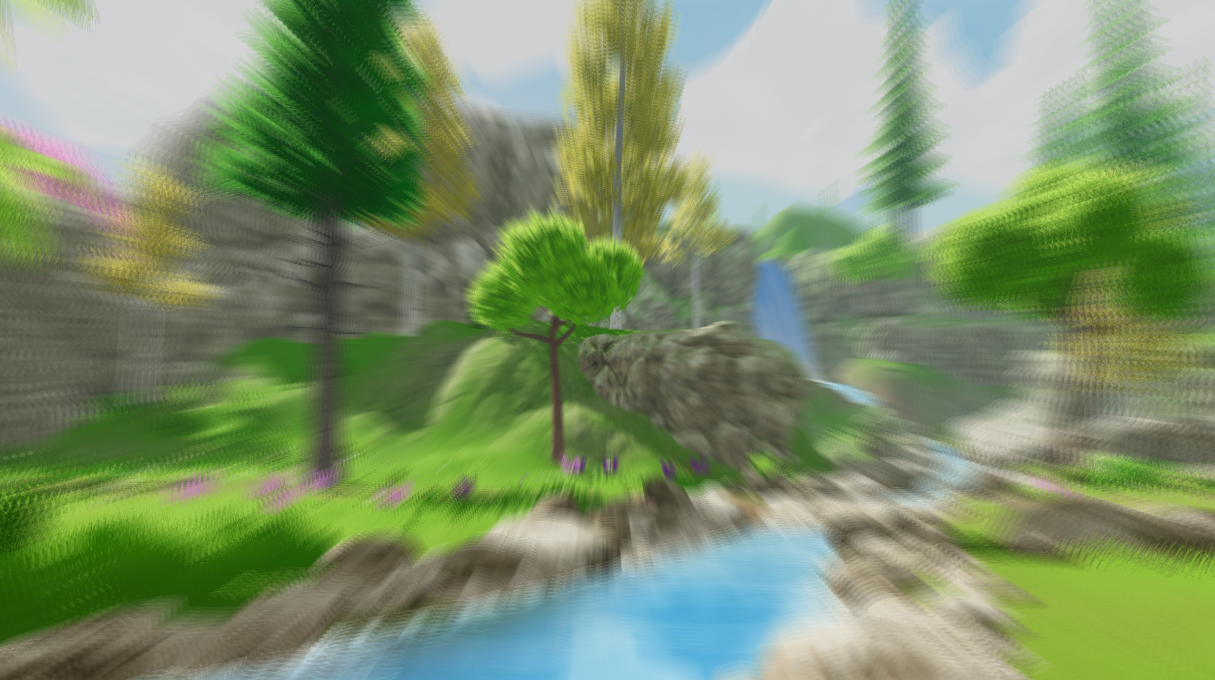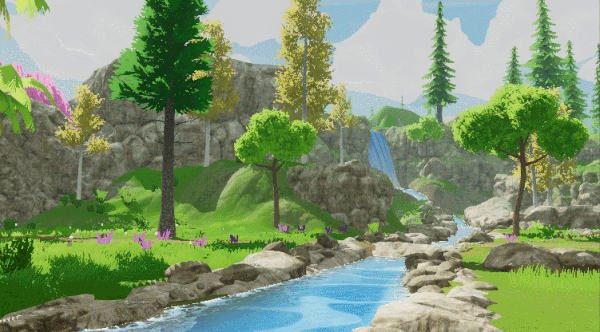# 十、方向模糊（Directional Blur）

``````float sinVal = (Mathf.Sin(settings.Angle) * settings.BlurRadius * 0.05f) / settings.Iteration;
float cosVal = (Mathf.Cos(settings.Angle) * settings.BlurRadius * 0.05f) / settings.Iteration;
``````

``````half4 DirectionalBlur(VaryingsDefault i)
{
half4 color = half4(0.0, 0.0, 0.0, 0.0);

for (int k = -_Iteration; k < _Iteration; k++)
{
color += SAMPLE_TEXTURE2D(_MainTex, sampler_MainTex, i.texcoord - _Direction * k);
}
half4 finalColor = color / (_Iteration * 2.0);

return finalColor;
}
``````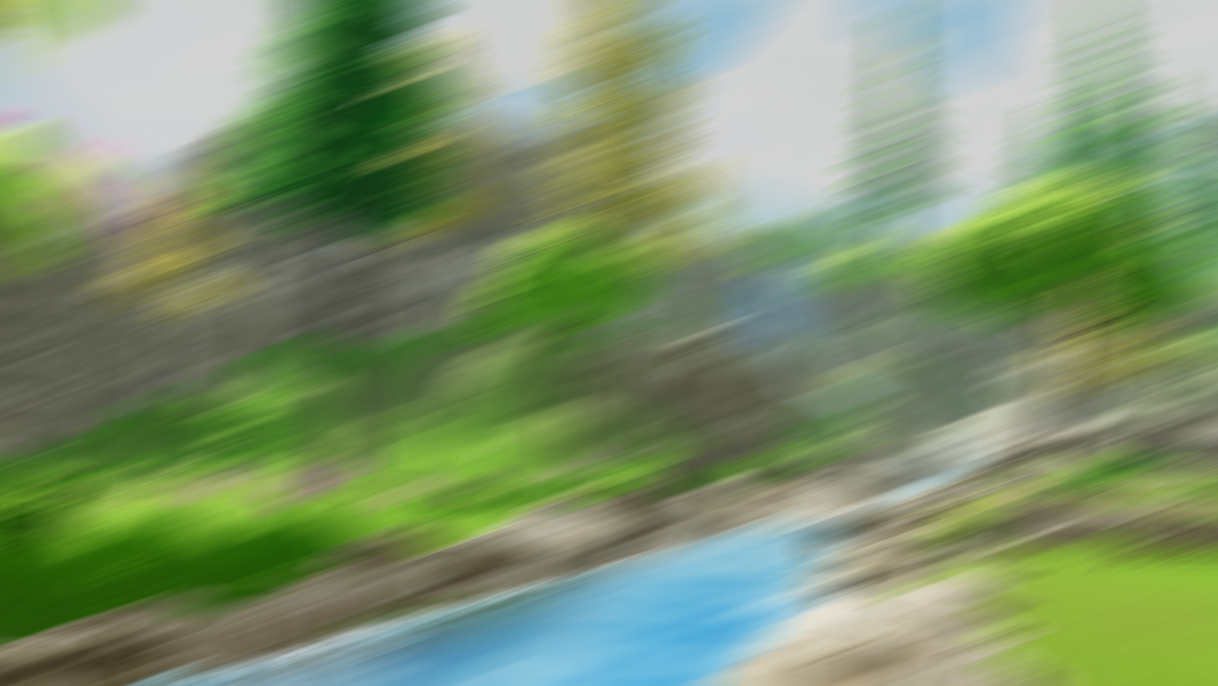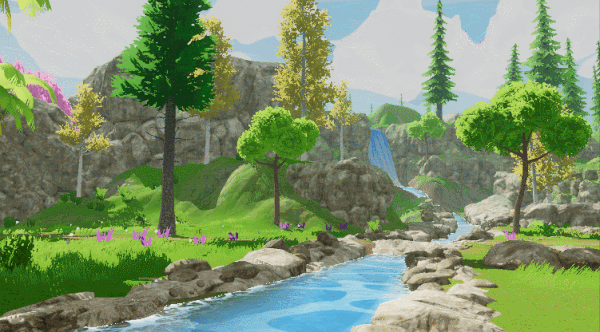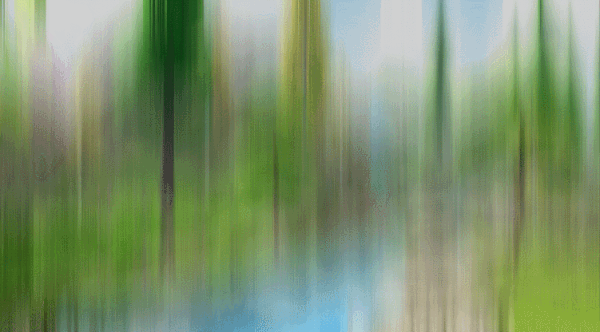# 总结The End.

# Reference

 GDC 2003, Frame Buffer Postprocessing Effects in DOUBLE-S.T.E.A.L (Wreckless)

 SIGGRAPH 2015, Bandwidth-Efficient Rendering

 SIGGRAPH 2001，Fast Image Convolutions

 Gonzalez R C, Woods R E. Digital image processing, 4th edn. ISBN: 9780133356724[J]. 2017.

0
0 收藏

### 作者的其它热门文章0 评论
0 收藏
0